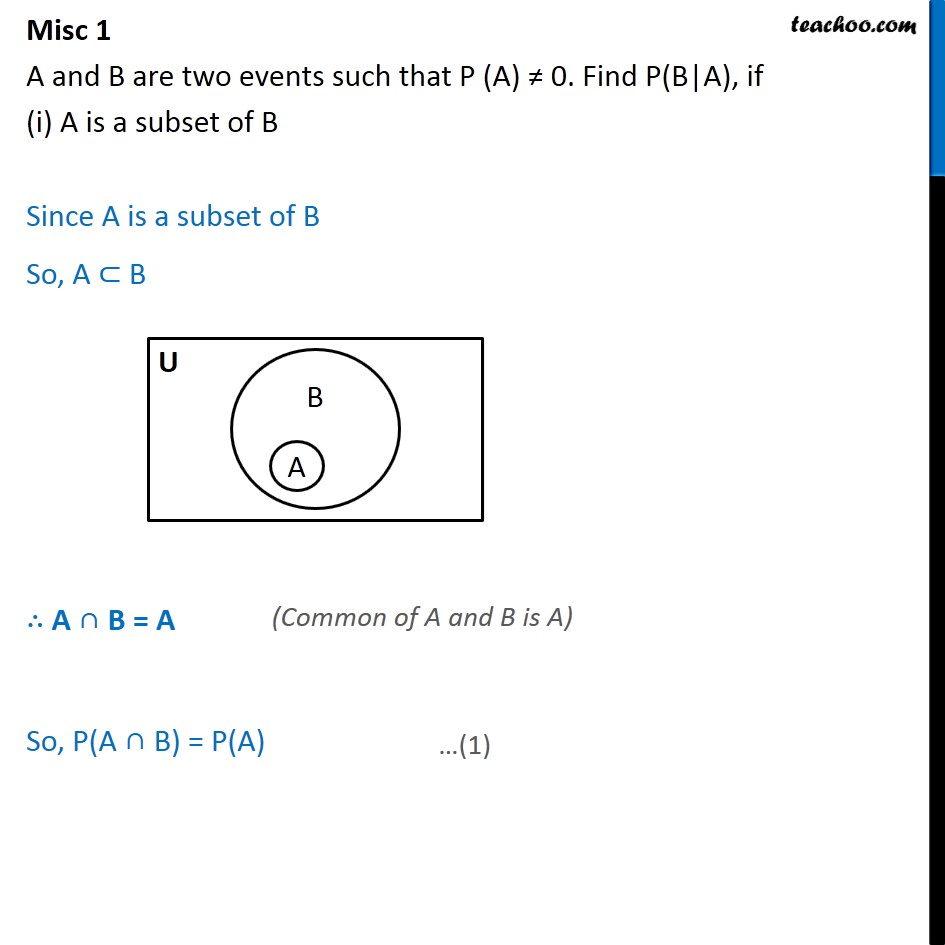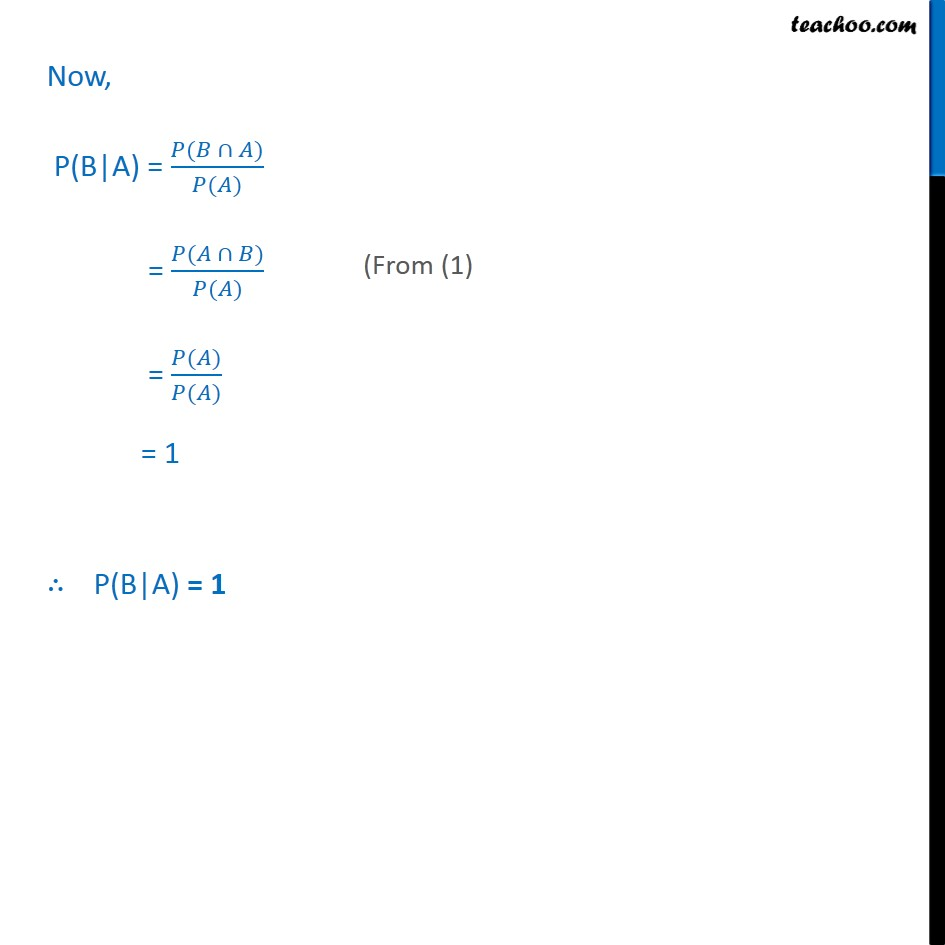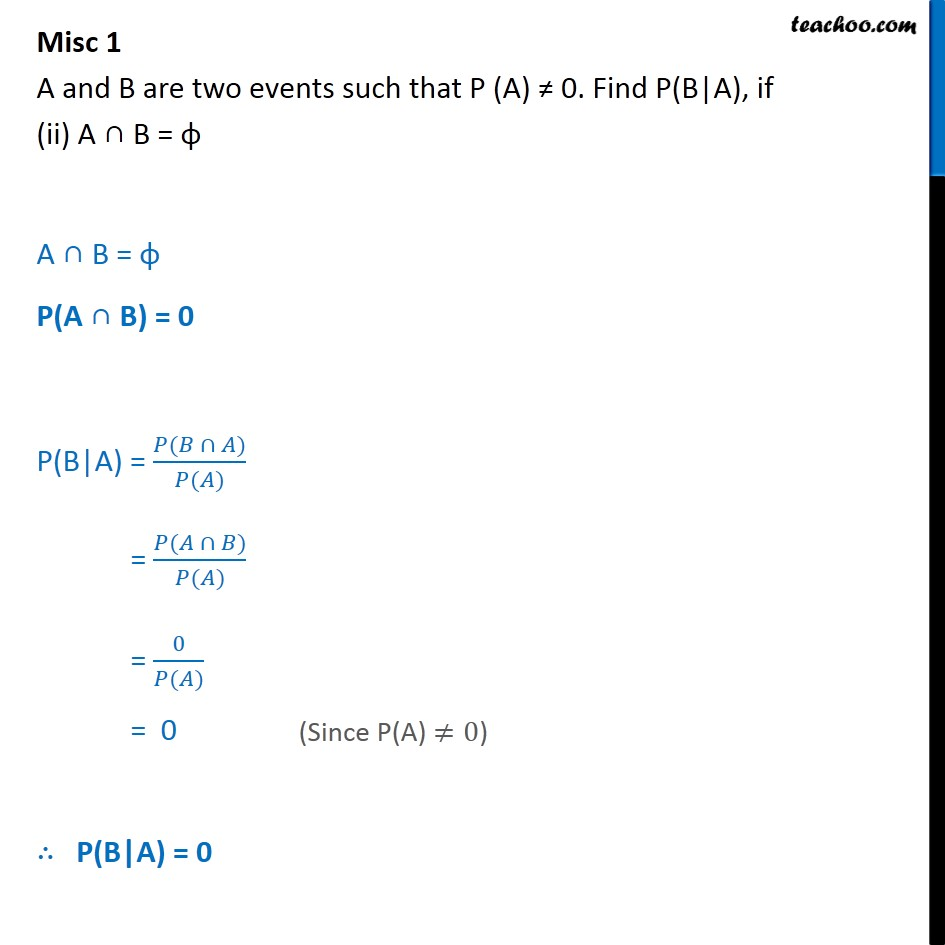Subscribe to our Youtube Channel - https://you.tube/teachoo

1. Chapter 13 Class 12 Probability
2. Serial order wise
3. Miscellaneous

Transcript

Misc 1 A and B are two events such that P (A) 0. Find P(B|A), if (i) A is a subset of B Since A is a subset of B So, A B A B = A So, P(A B) = P(A) Now, P(B|A) = ( ) ( ) = ( ) ( ) = ( ) ( ) = 1 P(B|A) = 1 Misc 1 A and B are two events such that P (A) 0. Find P(B|A), if (ii) A B = A B = P(A B) = 0 P(B|A) = ( ) ( ) = ( ) ( ) = 0 ( ) = 0 P(B|A) = 0

Miscellaneous